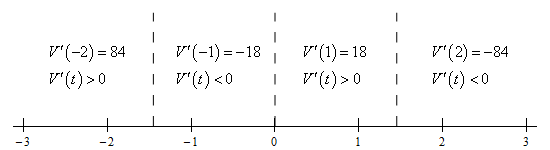Paul's Online Notes
Home / Calculus I / Derivatives / Product and Quotient Rule
Show Mobile Notice Show All Notes Hide All Notes
Mobile Notice
You appear to be on a device with a "narrow" screen width (i.e. you are probably on a mobile phone). Due to the nature of the mathematics on this site it is best views in landscape mode. If your device is not in landscape mode many of the equations will run off the side of your device (should be able to scroll to see them) and some of the menu items will be cut off due to the narrow screen width.

### Section 3.4 : Product and Quotient Rule

11. Determine where $$V\left( t \right) = \left( {4 - {t^2}} \right)\left( {1 + 5{t^2}} \right)$$ is increasing and decreasing.

Show All Steps Hide All Steps

Start Solution

We’ll first need the derivative, for which we will use the product rule, because we know that the derivative will give us the rate of change of the function. Here is the derivative.

$\require{bbox} \bbox[2pt,border:1px solid black]{{V'\left( t \right) = \left( { - 2t} \right)\left( {1 + 5{t^2}} \right) + \left( {4 - {t^2}} \right)\left( {10t} \right) = 38t - 20{t^3} = 2t\left( {19 - 10{t^2}} \right)}}$ Show Step 2

Next, we need to know where the function is not changing and so all we need to do is set the derivative equal to zero and solve. From the factored form of the derivative it is easy to see that the derivative will be zero at,

$\require{bbox} \bbox[2pt,border:1px solid black]{{t = 0 \hspace{0.5in} t = \pm \sqrt {\frac{{19}}{{10}}} = \pm 1.3784}}$ Show Step 3

To get the answer to this problem all we need to know is where the derivative is positive (and hence the function is increasing) or negative (and hence the function is decreasing). Because the derivative is continuous we know that the only place it can change sign is where the derivative is zero. So, as we did in this section a quick number line will give us the sign of the derivative for the various intervals.

Here is the number line for this problem.From this we get the following increasing/decreasing information.

\require{bbox} \bbox[2pt,border:1px solid black]{\begin{align*}{\mbox{Increasing :}} & \,\, - \infty < t < - \sqrt {\frac{{19}}{{10}}} ,\,\,\,\,\,\,0 < t < \sqrt {\frac{{19}}{{10}}} \\ {\mbox{Decreasing :}} & \,\, - \sqrt {\frac{{19}}{{10}}} < t < 0,\,\,\,\,\sqrt {\frac{{19}}{{10}}} < t < \infty \end{align*}}